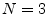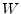Orthogonal Basis Computation Next  |  Prev  |  Up  |  Top  |  Index  |  JOS Index  |  JOS Pubs  |  JOS Home  |  Search

### Orthogonal Basis Computation

Matlab and Octave have a function orth() which will compute an orthonormal basis for a space given any set of vectors which span the space. In Matlab, e.g., we have the following help info:

```>> help orth
ORTH  Orthogonalization.
Q = orth(A) is an orthonormal basis for the range of A.
Q'*Q = I, the columns of Q span the same space as the
columns of A and the number of columns of Q is the rank
of A.

```

Below is an example of using orth() to orthonormalize a linearly independent basis set for:

```% Demonstration of the orth() function.
v1 = [1; 2; 3];  % our first basis vector (a column vector)
v2 = [1; -2; 3]; % a second, linearly independent vector
v1' * v2         % show that v1 is not orthogonal to v2

ans =
6

V = [v1,v2]      % Each column of V is one of our vectors

V =
1     1
2    -2
3     3

W = orth(V)  % Find an orthonormal basis for the same space

W =
0.2673    0.1690
0.5345   -0.8452
0.8018    0.5071

w1 = W(:,1)  % Break out the returned vectors

w1 =
0.2673
0.5345
0.8018

w2 = W(:,2)

w2 =
0.1690
-0.8452
0.5071

w1' * w2  % Check that w1 is orthogonal to w2

ans =
2.5723e-17

w1' * w1  % Also check that the new vectors are unit length

ans =
1

w2' * w2

ans =
1

W' * W   % faster way to do the above checks

ans =
1    0
0    1

% Construct some vector x in the space spanned by v1 and v2:
x = 2 * v1 - 3 * v2

x =
-1
10
-3

% Show that x is also some linear combination of w1 and w2:
c1 = x' * w1      % Coefficient of projection of x onto w1

c1 =
2.6726

c2 = x' * w2      % Coefficient of projection of x onto w2

c2 =
-10.1419

xw = c1 * w1 + c2 * w2  % Can we make x using w1 and w2?

xw =
-1
10
-3

error = x - xw

error = 1.0e-14 *

0.1332
0
0

norm(error)       % typical way to summarize a vector error

ans =
1.3323e-15

% It works! (to working precision, of course)
```

```% Construct a vector x NOT in the space spanned by v1 and v2:
y = [1; 0; 0];     % Almost anything we guess in 3D will work

%  Try to express y as a linear combination of w1 and w2:
c1 = y' * w1;      % Coefficient of projection of y onto w1
c2 = y' * w2;      % Coefficient of projection of y onto w2
yw = c1 * w1 + c2 * w2  % Can we make y using w1 and w2?
```
```yw =

0.1
0.0
0.3

yerror = y - yw

yerror =

0.9
0.0
-0.3

norm(yerror)

ans =
0.9487
```

While the error is not zero, it is the smallest possible error in the least squares sense. That is, yw is the optimal least-squares approximation to y in the space spanned by v1 and v2 (w1 and w2). In other words, norm(yerror) is less than or equal to norm(y-yw2) for any other vector yw2 made using a linear combination of v1 and v2. In yet other words, we obtain the optimal least squares approximation of y (which lives in 3D) in some subspace(a 2D subspace of 3D spanned by the columns of matrix W) by projecting y orthogonally onto the subspaceto get yw as above.

An important property of the optimal least-squares approximation is that the approximation error is orthogonal to the the subspace in which the approximation lies. Let's verify this:

```W' * yerror   % must be zero to working precision

ans = 1.0e-16 *

-0.2574
-0.0119
```

Next  |  Prev  |  Up  |  Top  |  Index  |  JOS Index  |  JOS Pubs  |  JOS Home  |  Search

[How to cite this work] [Order a printed hardcopy]